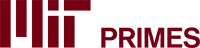#Yulia's Dream Research Papers

Yulia's Dream is a free math enrichment and research program for exceptional high school students (grades 10-11) from Ukraine.

## 2023 Research Papers

### 5) Dmytro Antonovych, Viktor Makozyuk, Vladyslav Tysiachnyi, Matching of frames of open Jacobi diagrams and chord diagrams (31 Jul 2023)

This paper is dedicated to the study of similarities between two objects that arise from the theory of Vassiliev invariants: open Jacobi diagrams and chord diagrams, which are uni-trivalent graphs with "orientation" structure and trivalent graphs with the structure of the chosen Hamilton cycle, respectively. We define a space of open Jacobi diagrams as the span of Jacobi diagrams modulo IHX and AS relations and a space of chord diagrams as a span of chord diagrams modulo 4T relations. We define two "frame" maps as operations from sets of chord and Jacobi diagrams to trivalent graphs. These operations act by forgetting about the structures in Jacobi and chord diagrams, making them trivalent graphs. Using these operations, we find the correspondence between the frames of elements of bases of spaces of Jacobi diagrams and chord diagrams, and formulate the "Frame matching" conjecture. As an intermediate step for proving the "Frame matching" conjecture, we prove a useful lemma, which states that any open Jacobi diagrams can be presented as a linear combination of special open Jacobi diagrams, which we called chord type Jacobi diagram. Finally, we wrote Python code to check the "Frame matching" conjecture; we provide an algorithm of how to use this code for checking the "Frame matching" conjecture.

### 4) Semen Andriets, Borys Holikov, Partial orderings of minors in the positive Grassmannian (24 Jul 2023)

In this paper, we research the partial order of minors of the positive Grassmannian (i.e. a space that parametrizes $k \times n$ matrices with all $k \times k$ minors positive) with a fixed maximal set of largest minors. This is connected to a combinatorial structure called a circuit graph. The maximal set of the largest minors corresponds to some cycle of length $n$ in the circuit graph, and then, abstractly, the further away in the graph some minor is from maximal the less its value. But this further is some non-trivial property called cubical distance, which be explained in the paper.

### 3) Alexander Borodin, Martin Leshko, Marina Spektrova, Combinatorial Hikita Conjecture (7 Jul 2023)

The Hikita conjecture is connected with geometry and representation theory. There are some generalisations, but all of them conjecture some isomorphism between algebras. Combinatorial objects we investigate, in some sense, enumerate their bases, and our general task, loosely speaking, is to find \textit{combinatorial} bijections. In particular, in this article, we will formulate and prove parabolic conjecture in combinatorial form. The advantage of this method over the previously known ones is the simplicity of the used objects, which means that this fact is accessible to a larger number of readers as well as it helps to understand the algebraic theorems better.

### 2) Ivan Bortnovskyi, Vadym Pashkovskyi, Exploration of the Grothendieck-Teichmueller ($GT$) shadows for the dihedral poset (4 Jul 2023)

Grothendieck-Teichmueller ($GT$) shadows are morphisms of the groupoid $GTSh$ and they may be thought of as approximations of elements of (the gentle version of) the Grothendieck-Teichmueller group $GTh$. The set $Ob(GTSh)$ of objects of $GTSh$ is the poset of certain finite index normal subgroups of the Artin braid group on 3 strands. In this note, we introduce a subposet $Dih$ of $Ob(GTSh)$, call it the dihedral poset, and investigate connected components of the groupoid $GTSh$ for elements of this poset. We prove that every $K \in Dih$ is the only object of its connected component $GTSh_{conn}(K)$ in the groupoid $GTSh$ (in particular, $GTSh_{conn}(K)$ is a finite group). We describe the set of morphisms of $GTSh_{conn}(K)$ explicitly and we show that, for every pair $N, K \in Dih$ such that $K \le N$, the natural map $GTSh_{conn}(K) \to GTSh_{conn}(N)$ is surjective.

### 1) Yehor Avdieiev (Yulia's Dream), Alexander Tsymbaliuk (Purdue University), Affine Standard Lyndon words: A-type (arXiv.org, 25 May 2023)

We generalize an algorithm of Leclerc describing explicitly the bijection of Lalonde-Ram from finite to affine Lie algebras. In type $A_n^{(1)}$, we compute all affine standard Lyndon words for any order of the simple roots, and establish some properties of the induced orders on the positive affine roots.

Support

To support Yulia’s Dream, we need your help! Please send a check to:
Massachusetts Institute of Technology
P. O. Box 412926
Boston, MA 02241-2926
Make the check out to "MIT" and in the memo field put "Yulia's Dream for PRIMES fund # 3653720."
Or, you can just click on the button below and choose "PRIMES Endowed Fund in Mathematics (3653720)" and designate your contribution “in memory of Yulia Zdanovska.”